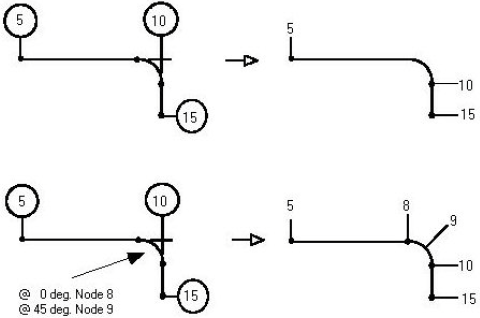# Bend Definition - CAESAR II - Reference Data

## CAESAR II Applications Guide (2019 Service Pack 1)

PPMProduct
CAESAR II
PPMCategory_custom
Reference Data
Version_CAESAR
11.0 (2019)

A bend is defined by the element entering the bend and the element leaving the bend. The bend curvature is always physically at the To end of the element entering the bend.

The input for the element leaving the bend must follow the element entering the bend. The bend angle is defined by these two elements. The default bend radius is 1-1/2 times the pipe nominal diameter (long radius), but it can be changed to any other value. When you specify a bend, two additional intermediate nodes are automatically generated--one at the 0º location and one at the bend midpoint (M).

For stress and displacement output, the To node of the element entering the bend is located geometrically at the far-point on the bend. The far-point is at the weld line of the bend, adjacent to the straight element leaving the bend. The 0º point on the bend is at the weld line of the bend, adjacent to the straight element entering the bend.

The From point on the element is located at the 0º point of the bend (and no 0º node point is generated) if the total length of the element as specified by DX, DY, and DZ is equal to:

R tan (b / 2)

Where b is the bend angle, and R is the bend radius of curvature to the bend centerline.

Nodes defined by the Angle and Node properties are placed at the given angle on the bend curvature. The angle starts with zero degrees at the near-point on the bend and goes to b degrees at the far-point of the bend. Angles are always entered in degrees. Entering the letter M as the angle designates the bend midpoint.

Nodes on the bend curvature cannot be placed closer together than the angle distance specified by Minimum Angle to Adjacent Bend in the Geometry category of the Tools > Configure/Setup command. This includes the spacing between the nodes on the bend curvature and the near- and far-points of the bend.

The minimum and maximum total bend angle is specified by the Minimum Allowable Bend Angle and Maximum Allowable Bend Angle properties, also in the Geometry category of the Tools > Configure Setup command.